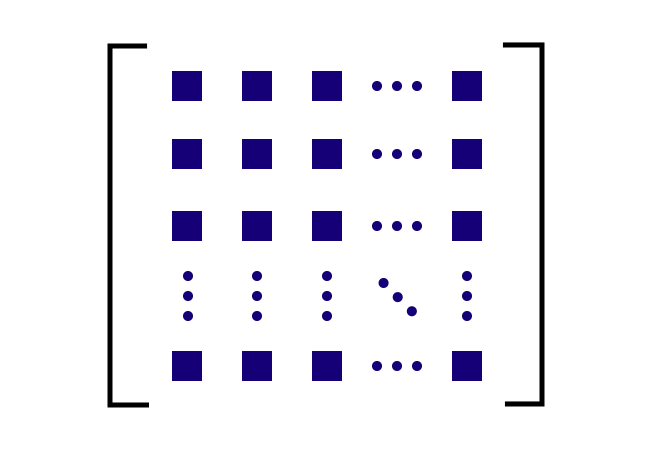# Diagonal elements of a Square Matrix

The elements that form a main diagonal in a square matrix are called the diagonal elements of a square matrix.

## Introduction

When the total number of elements in a row is equal to the total number of elements in a column, the arrangement of them in rows and columns forms a square matrix.The first element of the first row and the last element of the last row can be connected by a straight path diagonally. Similarly, an element from each row comes in this diagonal straight path.

Hence, the elements, which appear in the main diagonal of square matrix are the diagonal elements of the square matrix.

Let us learn how to identify the diagonal elements of matrix $M$ by identifying the principal diagonal or leading diagonal of the square matrix.

$M$ $\,=\,$ $\begin{bmatrix} \color{blue} e_{11} & e_{12} & e_{13} & \cdots & e_{1n} \\ e_{21} & \color{blue} e_{22} & e_{23} & \cdots & e_{2n} \\ e_{31} & e_{32} & \color{blue} e_{33} & \cdots & e_{3n} \\ \vdots & \vdots & \vdots & \color{blue}\ddots & \vdots \\ e_{n1} & e_{n2} & e_{n3} & \cdots & \color{blue} e_{nn} \\ \end{bmatrix}$

The square matrix $M$ has $n$ rows and $n$ columns. The elements $e_{11}$, $e_{22}$, $e_{33}$, $e_{44}$ and so on come in major diagonal region. Hence, they are called the diagonal elements of square matrix $M$.

### Example

Let $Q$ be a square matrix of order $4$. There are $16$ elements in this $4 \times 4$ square matrix. The sixteen elements are arranged in four rows and four columns.

$Q$ $\,=\,$ $\begin{bmatrix} \color{red} 0 & 5 & 7 & 9 \\ 8 & \color{red} 1 & 4 & 6 \\ 6 & 7 & \color{red} 2 & 9 \\ 4 & 4 & 8 & \color{red} 3 \\ \end{bmatrix}$

The elements $0$, $1$, $2$ and $3$ can be connected diagonally by a straight path. Hence, the elements are called the diagonal elements of square matrix.

Latest Math Topics
Apr 18, 2022
Apr 14, 2022

A best free mathematics education website for students, teachers and researchers.

###### Maths Topics

Learn each topic of the mathematics easily with understandable proofs and visual animation graphics.

###### Maths Problems

Learn how to solve the maths problems in different methods with understandable steps.

Learn solutions

###### Subscribe us

You can get the latest updates from us by following to our official page of Math Doubts in one of your favourite social media sites.